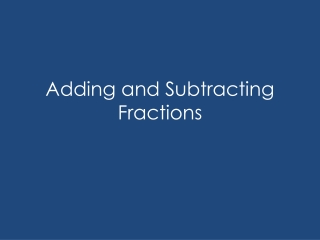DownloadDownload PresentationDownload Presentation- - - - - - - - - - - - - - - - - - - - - - - - - - - E N D - - - - - - - - - - - - - - - - - - - - - - - - - - -
##### Presentation Transcript

2. Review • What is a numerator? • What is a denominator? • What does a normal fraction look like? • What does a improper fraction look like? • What does a mixed number look like?

3. Like Fractions When fractions have the same denominator they are like fractions. You can add or subtract fractions when you have like fractions. These are all like fractions. 476325 5 5 5 5 5 5

4. Adding Like Fractions Steps: • Make sure that you have like fractions. • Add the numerators. • Write the sum over the denominator. • Write the answer in simplest form. 1 6 4 6 5 6 The answer is: 5 6

5. Subtracting Like Fractions Steps: • Make sure that you have like fractions. • Subtract the numerators. • Write the difference over the denominator. • Write the answer in simplest form. 7 8 3 8 4 8 The answer is: 1 2

6. Exercise #1 Add or subtract, simplify, use mixed numbers 1/5 21/4 • 1/5 + 2/5 = • 2/3 - 1/3 = • 3/4 + 2/4 = • 5/7 + 3/7 = • 3/2 - 1/2 = • 3/9 + 5/9 = • 2/8 - 2/8 = 3/5 8. 4/5 - 3/5 = 9. 7/8 + 3/8 = 10. 2/6 + 3/6= 11. 5/10 - 3/10= 12. 6/7 + 4/7 = 13. 9/12 + 3/12= 14. 2/7 - 1/7 = 15. 12/4 - 3/4 = 16. 3/6 + 9/6 = 17. 4/13 - 2/13 = 18. 7/9 - 1/9 = 19. 8/16 - 4/16 = 20. 2/3 + 5/3 = 1/3 12/8 2 5/6 11/4 2/13 12/7 1/5 8/9 1 13/7 1/4 8/9 1 21/3 0 1/7

7. Unlike Fractions Unlike fractions have different denominators. Adding or subtracting with unlike fractions isn’t that different from adding or subtracting like fractions. You just have to find what their denominators have in common. 2 3 3 4 5 6 5 8

8. Changing Unlike to Like Steps: Find a common multiple that will go into both denominators. Multiply the numerator and denominator by the same number so the denominator is the same as the common multiple. WHAT EVER YOU DO TO THE BOTTOM YOU HAVE TO DO TO THE TOP! 4 2 3 Now you’re ready to use these fractions for addition and subtraction! 3, 6, 9, 12, 15, 18 2 2 1 1 6 6 5 6 5 6, 12, 18, 24, 30, 36

9. Exercise #2 Add fractions, put them in simplest form 1/2 + 1/3 = 1/3 + 1/5 = 5/6 + 1/3 = 1/8 + 3/4 = 3/3 + 5/6 = 3/4 + 3/20 = 6/7 + 3/4 = 1/9 + 2/3 = 3/6 + 2/9 = 1/6 + 5/12 = 5/6 9/10 8/15 117/28 11/6 7/9 7/8 13/18 15/6 7/12

10. Exercise #3 Subtract fractions, put them in simplest form 1/5 - 2/5 = 1/4 - 1/6 = 7/8 - 2/3 = 4/5 - 3/10 = 8/9 - 1/3 = 3/5 - 1/3 = 1/3 - 1/6 = 9/10 - 2/5 = 2/3 - 1/2 = 4/5 - 1/3 = 1/10 4/15 1/12 1/6 5/24 1/2 1/2 1/6 7/15 5/9

11. Improper Fractions Improper fractions are easy! Just treat them as if they’re normal fractions. That’s it!

12. Unlike Improper Fractions 5/2 15/6 4/3 8/6 7/6 1 or 1/6

13. Mixed Numbers There are two ways to change mixed numbers so you can add or subtract. • Change the mixed number into an improper fraction. • Change just the fraction and leave the whole number alone.

14. Mixed Numbers 1 1/5 Steps: Add the fractions together. Add the whole numbers. 2 3/5 The answer is : 34/5 3 4/5

15. Unlike Mixed Numbers Steps: Change the unlike fractions to like fractions. Add the fractions. Add the whole numbers. If the fraction is improper, change it to mixed and add the whole numbers. 1 2 5/6 5/6 1/2 1/2 The answer is: 4 1/3 4 3 1 2/6 8/6 3/6

16. Exercise #4 Add or subtract, write in simplest form 1 3/8 - 11/8 = 2 2/5 + 1 1/5 = 1 7/8 – 1 6/8 = 3 4/7 + 2 3/7 = 4 8/9 - 2 1/9 = 2 2/5 + 1 1/10 = 3 1/4 – 2 1/2 = 5 2/3 + 3 1/2 = 3 5/6 – 1 3/5 = 6 1/4 - 3 2/3 = 1/4 31/2 3/4 33/5 1/8 91/6 27/30 6 10 27/9